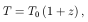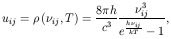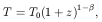3.1.4. POPRATIO

This is the main module that actually computes the rate coefficients for the processes described in section 2 (after reading the related atomic data from a separate input file) and then solves the system of equations of statistical equilibrium.

It begins defining a few useful constants to the evaluation of the rate coefficients and three new types:

• TABLE: this type is a table of points, Y vs. X;

• TCOLLISIONALDATA: this type encloses all necessary information pertaining a certain collisional process, such as the collision partner name, the collision rates, etc.,

• THLEVEL: this type encloses all necessary information pertaining some of the µ higher-lying levels described in section 2.2, such as the energy, statistical weight, transition probabilities, radiative rates, etc.

This module also declares several global variables that are visible to the main program. Table 1 shows the correspondence between most important variables and the mathematical quantities given in section 2.

 Variable Mathematical quantity E(i) Ei g(i) gi A(i,j) Aij B(i,j) Bij u(i,j) uij CD(k)%q(i,j) qkij gam(i,j)ij matrix(i,j) Mij indep(i) Ii X(i) Xi

We also have the following routines:

• SKIP_COMMENTS: subroutine used by INITPOPRATIO to skip the comments from the atomic data input file (see section 3.2 bellow);

• INITPOPRATIO: subroutine that reads in the atomic data values stored in the input file. It takes as input a unit number for the input file (defined via an open statement in the main program), the number of levels NBLEVELS to be taken into account in the calculation, and wavelength bounds to the radiation field intensities, LAMBDAI and LAMBDAF. The last two arguments are optional parameters, and if supplied by the user INITPOPRATIO will not load in any upper level connected to the ground term by multiplets whose wavelengths lie outside of this range (the default values are 0.0_WP and huge(1.0_WP)). INITPOPRATIO does not load into RAM memory all the data stored in the input file, only the necessary space to take into account the desired number of levels is allocated in run time. (See also section 3.2 below);

• FINISHPOPRATIO: subroutine that deallocates the dynamical variables allocated by INITPOPRATIO;

• POPULATIONRATIO: this is the key subroutine that sets the rates for all the processes and calculates the population ratios. It takes as input the kinetic temperature of the gas T (in K), the redshift Z, a vector N containing the volume densities (in cm-3) of the collision partners entered in the atomic data input file and two optional parameters: BETA and F.

The redshift is used to set the temperature of the Cosmic Microwave Background Radiation (CMBR), as given by the following relation predicted by the standard Big Bang cosmology (see, for instance, Kolb and Turner ):(19)

where T0 = 2.728 ± 0.002 K is the current value of the CMBR temperature [9, 10]. The effect of the CMBR blackbody radiation field is then included in the energy densities:(20)

where c is the speed of light. Alternatively, there are models that generalize the standard temperature law of the CMBR (19) by introducing a free parameter:(21)

whereis within the range 01. If this parameter is not entered via the optional parameter BETA, it is assumed zero. POPULATIONRATIO calls function TCMBR to evaluate the temperature of the CMBR, and next function PLANCK to evaluate its contribution to the energy densities. If the user does not wish to take the CMBR into account, the redshift Z should be entered with the value -1.0_WP.

POPULATIONRATIO also adds to the energy densities the contribution from a radiation field defined by the user in function URAD. The optional parameter F multiplies the radiation field defined in URAD by a constant factor f. If F is not entered, it is assumed 1.

• LTEDEV: this function returns the module of the largest relative deviation of the population of the lowest NL levels from the population expected from LTE conditions characterized by a temperature T_LTE (in K);

• URAD: this function returns the spectral energy density of a user-defined radiation field. The user may create his/her own subroutine and then call it in the body of this function.

• PLANCK: this function returns the spectral energy density of a blackbody radiation field (20). It first checks whether the argument of the exponential does not exceed the natural logarithm of the largest number in the mashine representation in order to avoid overflow (the spectral energy density is set to zero in case this happens).

• TCMBR: function that returns the temperature of the CMBR (21);

• RATE_EXTRAPOL: this function is called by POPULATIONRATIO whenever the kinetic temperature T lies outside the range allowed by some table of points of collisional rates entered in the atomic data input file (see section 3.2 below). The user must supply a subroutine to extrapolate the table of points and modify this function to call it, otherwise a warning message will be issued telling which particular table of points needs to be extrapolated. An analytical fitting to some transition may be implemented by entering an unphysical temperature range in the input file, so that whatever value of T is entered, POPULATIONRATIO will always call this function that may be used to call another function containing the fitting (such as CI_GAM and OI_PROTON, see example in section 3.2 below);

• CI_GAM: function that returns the Maxwellian averaged collision strengths for transitions - induced by collisions with electrons - involving the lowest five levels of C0 (based upon analytical fittings taken from the literature [12, 13]),

• OI_PROTON: function that returns the deexcitation rates by collisions with protons for transitions involving the lowest three levels of O0 (based upon analytical fittings taken from the literature ).